• MATLAB 】conv 函数介绍（卷积和多项式乘法
2021-04-24 21:14:56

conv

Convolution and polynomial multiplication

Syntax

w = conv(u,v)

w = conv(u,v,shape)

Description

w = conv(u，v)返回向量u和v的卷积。如果u和v是多项式系数的向量，则对它们进行卷积相当于将两个多项式相乘。

w = conv(u,v,shape) returns a subsection of the convolution, as specified by shape. For example, conv(u,v,'same') returns only the central part of the convolution, the same size as u, and conv(u,v,'valid') returns only the part of the convolution computed without the zero-padded edges.

w = conv(u，v，shape)返回卷积的子部分，由形状指定。

例如，conv(u，v，'same')仅返回卷积的中心部分，与u的大小相同，而conv(u，v，'valid')仅返回计算后的卷积部分而没有零填充边。

Polynomial Multiplication via Convolution

Create vectors u and v containing the coefficients of the polynomials x^2 + 1 and 2x + 7.

u = [1 0 1];

v = [2 7];

Use convolution to multiply the polynomials.

w = conv(u,v)

w = 1×4

2 7 2 7

w contains the polynomial coefficients for  2x^3 + 7x^2 + 2x + 7.

Vector Convolution

Create two vectors and convolve them.

u = [1 1 1];

v = [1 1 0 0 0 1 1];

w = conv(u,v)

w = 1×9

1 2 2 1 0 1 2 2 1

The length of w is length(u)+length(v)-1, which in this example is 9.

Central Part of Convolution

Create two vectors. Find the central part of the convolution of u and v that is the same size as u.

u = [-1 2 3 -2 0 1 2];

v = [2 4 -1 1];

w = conv(u,v,'same')

w = 1×7

15 5 -9 7 6 7 -1

w has a length of 7. The full convolution would be of length length(u)+length(v)-1, which in this example would be 10.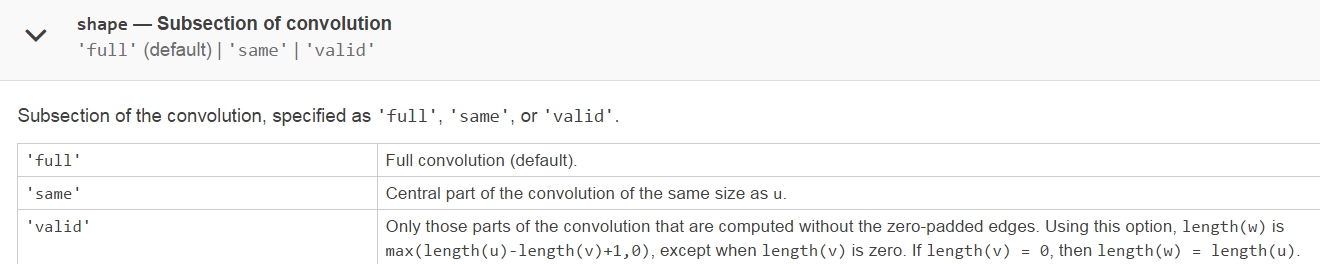更多相关内容
• ## MATLAB之多项式计算

万次阅读 多人点赞 2018-05-30 21:36:11
2、多项式乘法乘法用conv函数，例如：conv(p1,p2), p1、p2为两个多项式的系数向量，计算结果为p1*p2的新的向量的值。3、多项式除法： [Q,r]=deconv(p1,p2),多项式除法，Q返回p1/p2的商式，...

1、MATLAB里多项式的表示：

一个p阶的多项式可以用一个含有p+1个元素的向量表示，例如：2*x^2+1可以表示为[2 0 1]。这里的第一个元素为最高阶元素；

2、多项式的乘法：

乘法用conv函数，例如：conv(p1,p2), p1、p2为两个多项式的系数向量，计算结果为p1*p2的新的向量的值。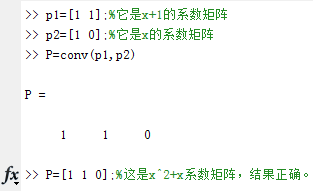3、多项式除法：

[Q,r]=deconv(p1,p2),多项式除法，Q返回p1/p2的商式，r返回p1/p2的余式。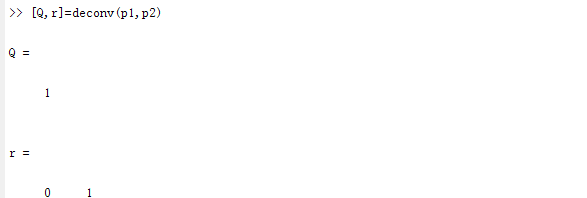注：p1与p2分别为x+1,x。

4、多项式求导函数

polyder(p);对多项式p求导;

polyder(p,q);对多项式p*q求导数；

[P,Q]=polyder(p,q);对多项式p/q求导数，求得导数的分子放入P中，分母放到Q中；

5、多项式的求值

polyval(p,x):代数多项式求值。

（1）如果x为一个标量，代表求p多项式在x点的值。

（2）如果x为一个向量或者矩阵，则对矩阵或者向量里面的每一个值求多项式的值。

polyvalm(p,x):矩阵多项式求值。

polyvalm与polyval的用法相同,但是要求x为方阵，求得结果是矩阵乘法。

6、多项式的求根：

x=roots(p),求多项式p的根。

如果已知根想重建多项式，可以用函数poly,利用方法

p=poly(x)。

展开全文• 接收多项式r1,r2(r1>=r2),以及多项式长度nums 返回其每一步的商组成的矩阵Q,以及gcd(r1,r2) Poly_Division(r1,r2,nums)： 接收多项式r1,r2(r1>=r2),以及多项式长度nums 返回商，及其余数 Poly_Multi(a,b,nums)...

# 一、解析

Poly_GCD(r1,r2,nums)：
接收多项式r1,r2(r1>=r2),以及多项式长度nums
返回其每一步的商组成的矩阵Q,以及gcd(r1,r2)

Poly_Division(r1,r2,nums)：
接收多项式r1,r2(r1>=r2),以及多项式长度nums
返回商，及其余数

Poly_Multi(a,b,nums)：
接收多项式a,b,以及多项式长度nums
返回乘积

Poly_Multi_Eye(a,b,nums)：
接收多项式a，仅有一个系数为1的多项式b,以及多项式长度nums
返回乘积

# 二、思路

函数Poly_GCD(r1,r2,nums)返回其商的矩阵Q，以及最大公因式gcd
X初始迭代值为gcd，Y初始迭代值为任意多项式
设X初始迭代值为gcd，Y初始迭代值为0
迭代方程如下：
Next_X=Y
Next_Y=X-Q_i*Y
单击查看迭代方程证明
最终输出X,Y迭代值

# 三、演示图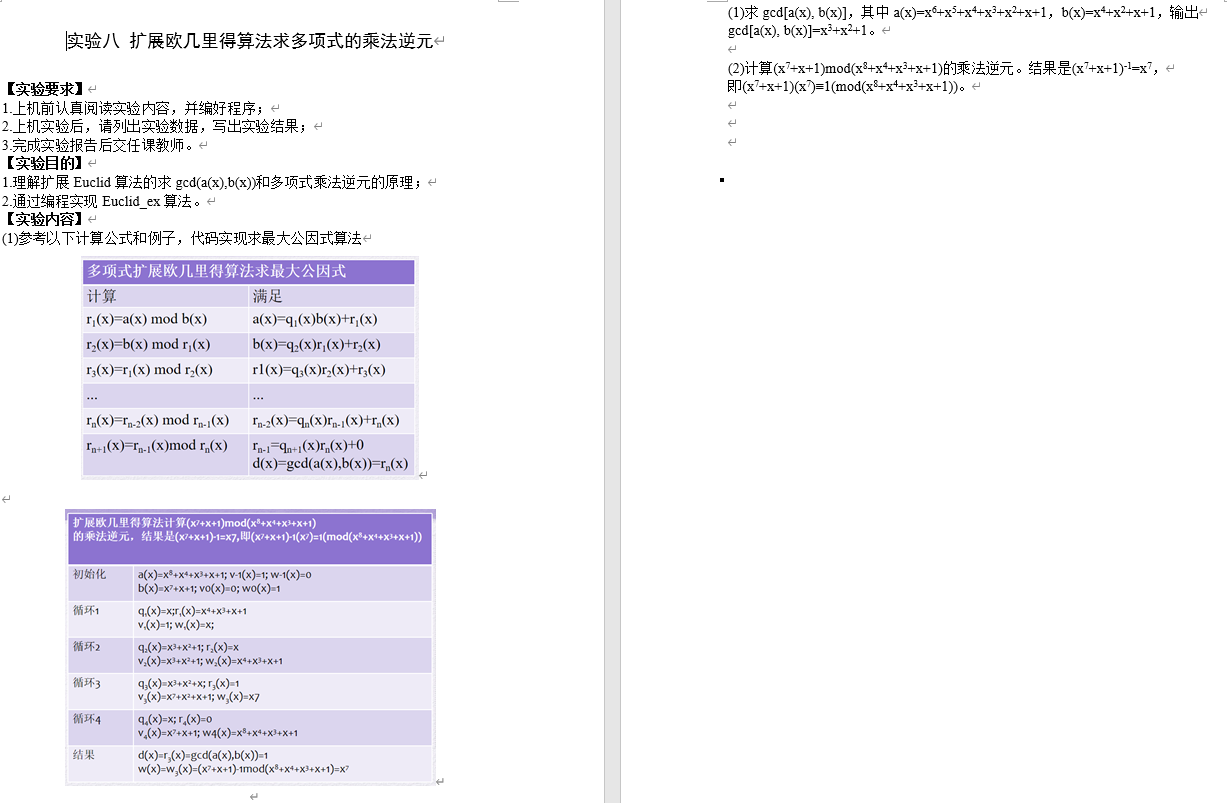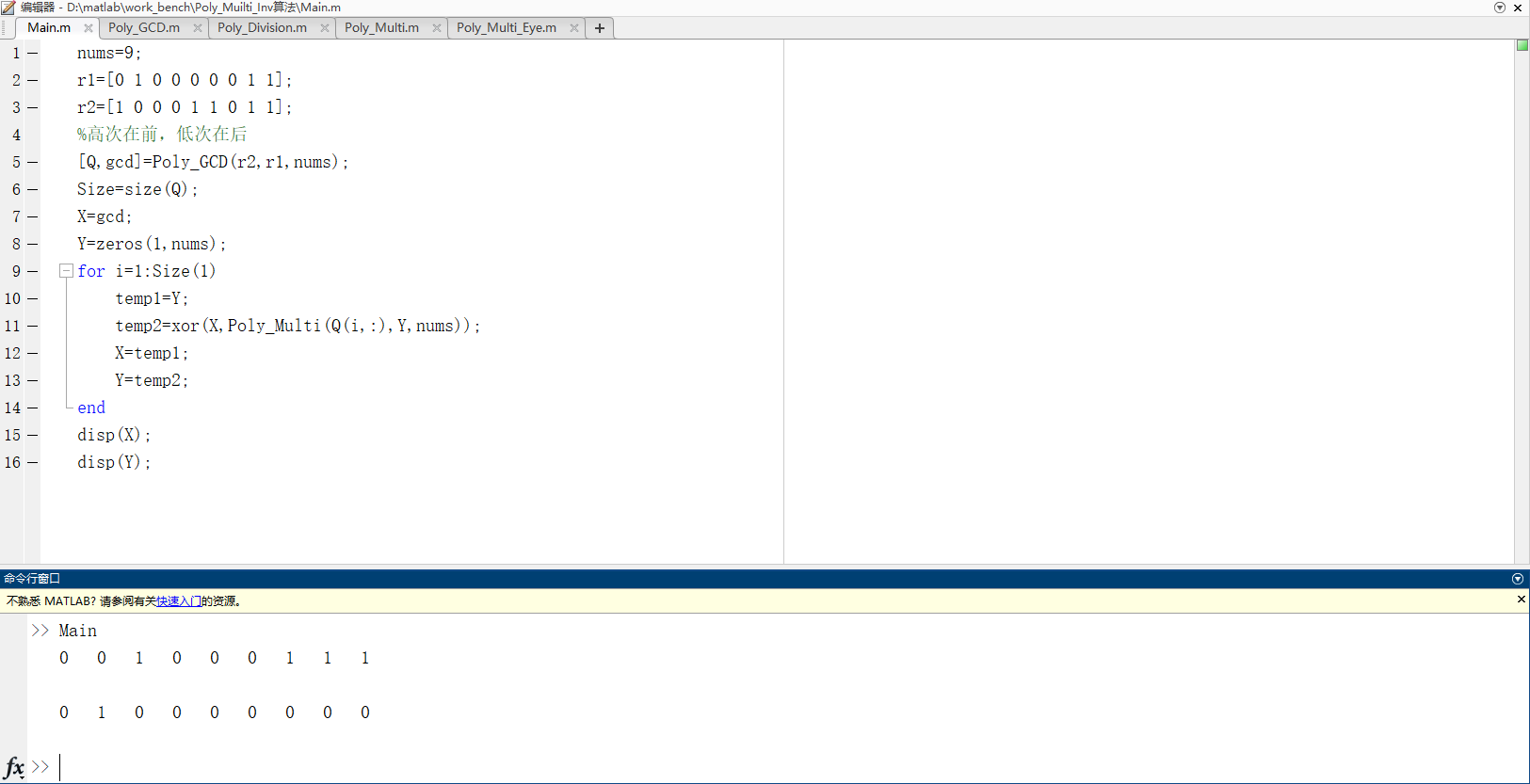# 四、代码

## 1、Main

nums=9;
r1=[0 1 0 0 0 0 0 1 1];
r2=[1 0 0 0 1 1 0 1 1];
%高次在前，低次在后
[Q,gcd]=Poly_GCD(r2,r1,nums);
Size=size(Q);
X=gcd;
Y=zeros(1,nums);
for i=1:Size(1)
temp1=Y;
temp2=xor(X,Poly_Multi(Q(i,:),Y,nums));
X=temp1;
Y=temp2;
end
disp(X);
disp(Y);


## 2、Poly_GCD

function [Q,r2] = Poly_GCD(r1,r2,nums)
Q=[];
[q,r3]=Poly_Division(r1,r2,nums);
Q=[q;Q];
while(~(max(r3)==0))
r1=r2;
r2=r3;
[q,r3]=Poly_Division(r1,r2,nums);
Q=[q;Q];
end
end


## 3、Poly_Division

function [Q,r3] = Poly_Division(r1,r2,nums)
Q=zeros(1,nums);
temp1=find(r1==1);
temp2=find(r2==1);
temp3=temp2(1)-temp1(1);
while(temp3>=0)
q1=zeros(1,nums);
q1(1,nums-temp3)=1;
Q=Q+q1;
Poly_Multi(r2,q1,nums);
r3=xor(r1,Poly_Multi(r2,q1,nums));
if(max(r3)==0)
break;
end
r1=r3;
temp1=find(r1==1);
temp3=temp2(1)-temp1(1);
end
end


## 4、Poly_Multi

function [ret] = Poly_Multi(a,b,nums)
temp1=find(b==1);
Size=size(temp1);
ret=zeros(1,nums);
for i=1:Size(2)
temp2=zeros(1,nums);
temp2(1,temp1(i))=1;
ret=xor(ret,Poly_Multi_Eye(a,temp2,nums));
end
end


## 5、Poly_Multi_Eye

function [ret] = Poly_Multi_Eye(a,b,nums)
temp1=find(b==1);
for i=1:nums-temp1(1)
a(1,1:nums-1)=a(1,2:nums);
a(1,nums)=0;
end
ret=a;
end


展开全文算法
• 对于数据数组 x 的任何结构，多项式是使用精确的 l/2 乘法和加法计算的。 多项式系数对于中度/高度可能非常大，从而导致精度降低。 对于这些情况，最好使用递归公式。 第二个可选输出基于最大多项式系数估计误差。...
• % 使用多项式乘法进行计算机双线性变换% 输入是 H(s) 降序多项式% 输出是降序多项式中的 H(z) % % 用法：[c,d] = mBilinear(a,b,Fs); % with a: 按 H(s) 分子降序排列的向量% b：按 H(s) 分子降序排列的向量% c: H(z...
• % 使用霍纳方法以嵌套形式计算多项式的值 % 输入：多项式的阶d， % d+1个系数构成的数组c（第一个元素为常数项）, % x坐标需要进行求值的x位置， % 如果需要的话，还有d个基点构成的数组b % 输出：多项式在x点对应...

计算机求解多项式需要多次乘法，为了减少乘法次数可使用嵌套乘法。

如若多项式为：

则可使用嵌套法展开为：

### matlab实现：

% 嵌套乘法
% 使用霍纳方法以嵌套形式计算多项式的值
% 输入：多项式的阶d，
%       d+1个系数构成的数组c（第一个元素为常数项）,
%       x坐标需要进行求值的x位置，
%       如果需要的话，还有d个基点构成的数组b
% 输出：多项式在x点对应的值y
function y = nest(d,c,x,b)
if nargin<4
b=zeros(d,1);
end
y=c(d+1);
for i=d:-1:1
y=y.*(x-b(i))+c(i);
end`

可以验证此算法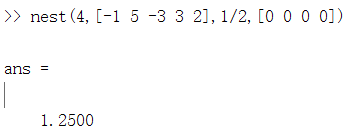若所有基点为0，可使用如下缩减形式的命令：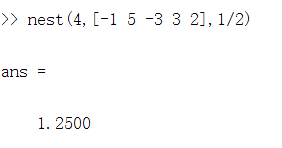使用该命令可以得到同样的结果，这是由于在nest.m中对于输入参数的设置，如果输入的参数个数小于4，基点被自动设置为0

由于matlab对于向量表达的无缝处理，嵌套命令可以同时估计一组x对应的多项式的值，如下：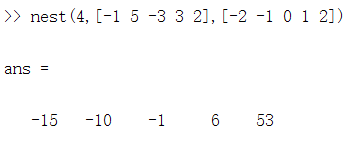本文参考自数值计算（原书第2版）

展开全文• 文章目录0 前言1 多项式的...本文是科学计算MATLAB语言课程的第5章第2小结的学习笔记，通过查阅本文，可以轻松掌握利用MATLAB进行多项式的四则运算（加减乘除）、求导、求值、求根等，Enjoy your read...windows 矩阵
• 资源名：ar模型_多项式乘法_潮流计算_NSGA_场景削减_MATLAB编程代码 资源类型：matlab项目全套源码 源码说明： 全部项目源码都是经过测试校正后百分百成功运行的，如果您下载后不能运行可联系我进行指导或者更换。 ...
• 还使用多项式乘法算法来测试基本的DFT算法。 在O（n log n）多项式乘法中的应用 DFT算法可以方便地应用于多项式乘法。 结果是O（n log n）多项式乘法算法。 实现是。 此应用程序还用于测试FFT的实现，测试用例为。 ...
• 多项式计算 roots ployval MATLAB
• ,matlab中相加的多项式变为相乘的多项式用什么命令matlab计算多项式相乘？？,matlab中相加的多项式变为相乘的多项式用什么命令匿名网友:本质上是一样的。向量卷积和多项式函数间的卷积从算法这个角度上，是一样的，...
• matlab : R2018a 64bit      OS : Windows 10 x64 typesetting : Markdown    blog : my.oschina.net/zhichengjiu    gitee : gitee.com/zhichengjiu   code clear clc a=[1 2] b=[1 2] c=...
• 1、多项式乘法 conv conv(A,B) 说明：A、B表示降幂排列的多项式系数 2、多项式求根 roots 3、由根创建多项式 poly 4、求多项式在给定点的值 polyval
• matlab中可以用数组表示： p=[2 -3 0 -1] 多项式的根： roots(p) 多项式的创建：poly函数的运用 p=[2 -3 0 -1]；r=roots(p)； ss=poly(r) ss 只知道多项式的零点，可以用poly函数来创建多项式的...数学
• 使用matlab使用嵌套乘法计算多项式，有效提高计算效率
• 一、Matlab 卷积和多项式乘法 conv 函数、 二、使用 matlab 代码求卷积并绘图数字信号处理 卷积
• MATLAB 中，n次多项式用一个长度为 n+1 的行向量表示。如有n次多项式： 则在 MATLAB 中， p(x) 表示为向量形式：[an,a(n-1),a(n-2),a(n-3),……,a0] 在 MATLAB 中创建多项式向量时，要注意三点： （1）多项式...
• 配方, 如何用 matlab 进行多项式运算 (1) 合并同类项 syms 表达式中包含的变量 collect(表达式,指定的变量) (2)因式分解 syms 表达式中包含的变量 factor(表达式)......配方, 如何用 matlab 进行多项式运算 (1) 合并...
• 这种排序的使用允许使用numpy.fft在多项式之间执行乘法。 总的来说，已经付出了很大的努力来加快所有运算的速度，从而使该模块适合于处理非常大的（几万个）GF2 [x]多项式，例如编码理论中所要求的多项式。 与numpy....
• ## MATLAB多项式计算

千次阅读 2020-08-23 16:45:02
MATLAB多项式计算 在MATLAB中，n次多项式用一个长度为n+1的行向量表示（因为是n个幂次项系数加上一个常数项），缺少的幂次项系数为0。 例如 n次多项式： P(x)=anxn+an-1xn-1+an-2xn-2+…+a1x+a0 表示为： [an,an-1,...
• 以C为例,理论可以借鉴!!不准备多写，这些东西网上可以搜出一箩筐来。高精运算库的构想，是可以快速的应付巨数的运算，包括加，减，乘，除等。比如现在的int是16字节，long...乘法如果直接像手工算一样做，复杂度是O(...
• 文章目录一、解析/思路二、代码三、Main1、...Func1：接收多项式f与拆分的多项式gi，返回fgi的结果 Func2：接收分步相乘的结果T，返回异或结果 二、代码 三、Main f=[0 1 0 0 1 1 0 1]; g=[1 0 0 1 1 0 0 1]; m=[0 0 0矩阵
• function [s1,n]=polydegree(p,x) init=0; p0=p; while ~isreal(p)|p~=0 p=diff(p,x); init=init+1; end init=init-1; s=sym([]);...s(i)=diff(p0,x,init-i+1)/(factorial(init-i+1));...n=length(s1).
• 1.多项式求根在MATLAB中求取多项式的根用roots函数。函数:roots功能:一元高次方程求解。语法:roots(c)说明:返回一个列向量，其元素为多项式c的解。例:求方程x3-8x2+6x–30=0的解。c = [0 -8 6 30]; %注意需包含所有...
• matlab实验多项式运算数学实验 Matlab 多项式运算与代数方程求解器 Matlab 多项式运算 Matlab多项式的表示方法 在 Matlab多项式是用它的系数向量来表示的。 例：2x3-x2+3 [2,-1,0,3] 特别注意：系数中的零是不...
• 一、多项式的表示方法1.系数向量直接输入法例1：>> p = [1 -5 6 -33];>> poly2sym(p)ans =x^3 - 5*x^2 + 6*x – 332.特征多项式输入法例2：>> a = [1 2 3;2 3 4;3 4 5];>> p1 = poly(a)p1 =...
• 1、多项式的表示 在MATLAB中创建多项式向量时，注意三点: ...(2)多项式乘法 conv (P1,P2):多项式相乘函数。 在这里，P1、P2是两个多项式系数向量。 (3）多项式除法 [Q,r]=deconv(P1,P2):多项式相除函数。 其中，Q返.
• conv( )多项式乘法 deconv( )多项式除法 [Q,r]=deconv(P1,P2):多项式相除函数。 其中，Q返回多项式P1除以P2的商式，r返回P1除以P2的余式。Q和r仍是多项式系数向量。 deconv是conv的逆函数，因此有P1=conv(Q, P2)+r...
• ## MATLAB多项式

千次阅读 2021-04-18 04:11:39
多项式的表达式MATLAB用一个行向量来表示多项式，此行向量就是将幂指数降序排列之后多项式各项的系数。例如，考虑下面的表达式：这就是Wallis在他第一次在法国科学院提出牛顿法的时候所用的多项式。在MATLAB中，该......

# matlab计算多项式乘法matlab 订阅